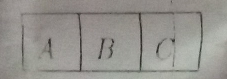Name the parts where the strength of the magnetic field is :

(i) maximum ">

# The given magnet is divided into three parts $A$, $B$, and $C$.Name the parts where the strength of the magnetic field is :(i) maximum

$(i)$. In regions A and C magnetic field is maximum.

$(ii)$. In region B magnetic field is minimum.

As it is clear that $A$ and $C$ have the maximum number of magnetic field lines which determine the intensity of the magnetic field because $A$ and $C$ are magnetic poles while Part $B$ lies in the center of the magnet, so it has no magnetic field lines. So the intensity of the magnetic field near B is almost zero.

Updated on: 10-Oct-2022

34 Views